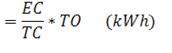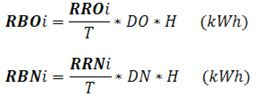ELECTRICITY CHARGE CALCULATION IN MONTHS WITH CHARGE RATE

1. Basis for charge calculation:

- The actual electricity consumption during the meter reading period;

- The number of days of actual electricity consumption (the days between two meter readings);

- Electricity charge rate

2. Method of calculation of electricity consumption  amounts that are subject to the old and new prices:

- For customers having their meter reading recorded at the turning point to the period of the new price: The electricity consumption that is subject to the old price is calculated as the difference between the meter reading recorded at the beginning of the period of the new price and the meter reading recorded in the previous period. The electricity consumption subject to the new price is based on the meter reading recorded at the beginning of the new price and the meter reading of the current meter reading period;

- For customers signing electricity purchase contract for residential consumption purposes and customers who do not have the meter reading recorded at the turning point to the period of the new price, the electricity consumption amounts that are subject to the old and new prices are calculated as follows:

Electricity consumption subject to the old priceElectricity consumption subject to the new price

= EC - electricity consumption subject to the old price

In which: EC is the electricity consumption in the current meter reading period for billing;

TC is the actual time of consumption calculated as the number of days from the previous meter reading day to the current billing period meter reading day (unit: days);

TO is the time of old price calculated as the number of days from the previous meter reading day to the day from which the new price is applicable (unit: days)

3. Method of calculation of household electricity consumption rangesIn which: RBOi is the Range of electricity consumption number i which is used for billing at the old pricing (kWh);

RBNi is the Range of electricity consumption number i which is used for Billing at the New pricing (kWh);

RROi is the Range of electricity consumption number i which is Regulated by the Old pricing (kWh);

RRNi is the Range of electricity consumption number i which is Regulated by the New pricing (kWh);

T is the number of days according to the calendar of the preceding month (unit: days);

DO is the number of days that are subject to the old price (from the meter reading day of the preceding month to the day from which the new price is applicable) (unit: days);

DN is the number of days that are subject to the new price (from the day from which the new price is applicable to the meter reading day for the current billing period) (unit: days).

H is the number of households which consume electricity collectively.

The values of RBOi and RBNi are arithmetically rounded.

• 10/12/2016 03:43